Next: Matrix decompositions and latent Up: Hierarchical clustering Previous: References and further reading   Contents   Index

# Exercises

Exercises.

• A single-link clustering can also be computed from the minimum spanning tree of a graph. The minimum spanning tree connects the vertices of a graph at the smallest possible cost, where cost is defined as the sum over all edges of the graph. In our case the cost of an edge is the distance between two documents. Show that if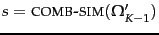are the costs of the edges of a minimum spanning tree, then these edges correspond to the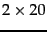merges in constructing a single-link clustering.

• Show that single-link clustering is best-merge persistent and that GAAC and centroid clustering are not best-merge persistent.

1. Consider running 2-means clustering on a collection with documents from two different languages. What result would you expect?
2. Would you expect the same result when running an HAC algorithm?

• Download Reuters-21578. Keep only documents that are in the classes crude, interest, and grain. Discard documents that are members of more than one of these three classes. Compute a (i) single-link, (ii) complete-link, (iii) GAAC, (iv) centroid clustering of the documents. (v) Cut each dendrogram at the second branch from the top to obtain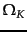clusters. Compute the Rand index for each of the 4 clusterings. Which clustering method performs best?

• Suppose a run of HAC finds the clustering with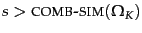to have the highest value on some prechosen goodness measure of clustering. Have we found the highest-value clustering among all clusterings with?

• Consider the task of producing a single-link clustering of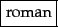points on a line:

Show that we only need to compute a total of aboutsimilarities. What is the overall complexity of single-link clustering for a set of points on a line?

• Prove that single-link, complete-link, and group-average clustering are monotonic in the sense defined on page 17.1 .

• Forpoints, there are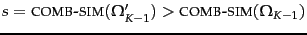different flat clusterings into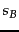clusters (Section 16.2 , page 16.2.1 ). What is the number of different hierarchical clusterings (or dendrograms) ofdocuments? Are there more flat clusterings or more hierarchical clusterings for givenand?Next: Matrix decompositions and latent Up: Hierarchical clustering Previous: References and further reading   Contents   Index